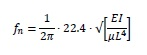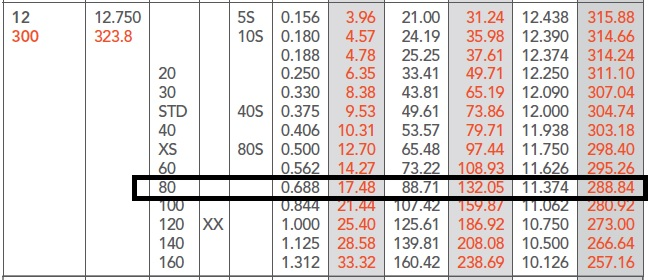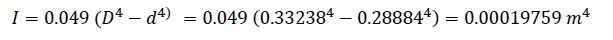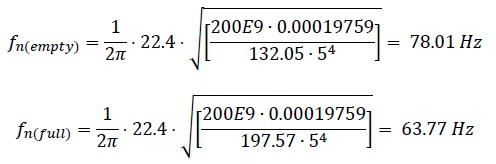16 Sep, 2016

# Piping Vibration Part 1: Natural Frequency of a Pipe

So there you are, sitting in your office having a well-earned cup of coffee.  The phone rings and on the other end is an irate production supervisor screaming that the plant is about to explode because that new section of pipe you installed last week is galloping up and down and vast amounts of natural gas will be released into the plant when the pipe breaks.  You calm him down and you arrange to take the section of pipe out of service (quickly).  Now everything is safe and you have to figure out what went wrong.

Part 1 Natural Frequency of a Pipe

Why do pipes gallop?

Maybe galloping isn’t the right word; I would prefer to say the pipe is vibrating.  But why is it vibrating so much?  One word – resonance.

If you seat a child on a swing and you give a gentle nudge to the swing every time the child swings back towards you, you will quickly be able to get the swing higher and higher.  You only need a very small force because you waited until the swing had reached its limit of movement (the limit of the swing) and only then did you give that gentle push.  But you did it EVERY time the swing moved back towards you.  A couple of interesting things are happening here.

(1) You are pushing at exactly the same frequency as the frequency at which the child is swinging. Don’t forget that if you are troubleshooting a pipe vibration problem the natural frequency will depend not only on the steel of the pipe but also the mass of the fluid inside.

(2) You are pushing at the same position in the swing cycle – in other words you have “locked phase” with the swing.

With these two “interesting things” it means you are in RESONANCE with the swing.  When a pipe is vibrating heavily it is almost always because there is a resonance issue.  The swing has a natural frequency because it has a period of oscillation that depends on the mass of the swing and the length of the pendulum.  This is the reason why a pendulum is used on a clock.  If you can work out the period of the cycle back and forth, then you know the frequency.

If the time period of oscillation is one second then the frequency is one per second or 1Hz.  If the time period is half a second then the frequency is 2Hz and so on.  By the same reasoning the pipe has a natural period of oscillation and so it has a natural frequency.  The natural frequency of the pipe depends on its stiffness and its mass; the stiffer the pipe the higher the frequency, the more mass the pipe (including contents) has, the lower the natural frequency.

To calculate the natural frequency of a pipe with rigid supports use the following formula:Where:

f = natural frequency of the pipe (Hz)

E = Young’s modulus of elasticity (200GPa or 30E6psi for steel – approximately but close enough)

I = 4th polar moment of inertia for the pipe (0.049*[OD^4-ID^4]) in inches or metres

µ = mass per unit length of the pipe (remember to include the mass of the fluid) lbs/inch or kg/m

L = distance between pipe supports (inches or metres)

Let’s find the natural frequency of a 12” (300mm) pipe made of A-106 GrB schedule 80 that is first empty and then filled with water.  I’ll use SI units to make the math easier.  Pipe supports are 5m apart.

Given:

Pipe OD = 323.8 mm, Pipe ID = 288.84 mm,

Mass/length empty= 132.05 kg/m,

Mass/length full = 132.05 + π(0.28884)^2/4 * 1000 = 197.57 kg/m E = 200 x 10^9 PaCalculate INatural Frequency:Now you know the natural frequency of the pipe you ask your vibration techs to take a vibration measurement on the pipe when it’s in operation.  If the frequency is the same as your calculated frequency, then the pipe has a resonance problem and the next step is to identify what is the force that is exciting the natural frequency.

Check out the next STEP - STEP 2 - Calculate VIV Vortex Induced Vibration affecting the pipe.

For more information and to get a fuller understanding of piping issues attend our class ME-41 "Piping Systems - Mechanical Design & Specification" running in Dubai in November.